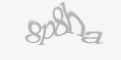Blog

360dissertations understands how tough Masters level projects can be. The standard of a post graduate course is higher than a bachelor’s degree.

Ordinal logistic regression, an extension of simple logistic regression test, is a statistical technique used to predict the relationship the relationship between an ordinal dependent variable and one or more independent variables. Commonly known as ordinal regression test, this statistical technique lets you determine if the independent variables have statistical significant effect on the dependent variables.

Examples,

A researcher investigates the factors that influence the decision of applying to the post graduate degree. Students were questioned if they are very likely, likely or somewhat likely to apply to the post graduate degree. The outcome of the research has three variables. (1) the institution is private or public, (2) present GPA and (3) educational status of the parents. The researcher believed that the difference between likely, somewhat likely and very likely are not equal.

Ordinal regression can be used to determine the reaction of patients to drug dosage. The outcomes can be classified as severe, moderate, mild or none. Here, it was believed that the difference between mild and moderate is not easy to quantify and the difference between mild, moderate, severe is not equal.

Performing ordinal regression involves checking for data and ensuring they hold good for all the assumptions that are needed to obtain a valid result. The assumptions of ordinal logistic regression model are as follows.

• The dependent variable must be measured at an ordinal level.

• One or more independent variables are ordinal, categorical or continuous. The ordinal independent variables must be treated as either continuous or categorical and not as an ordinal variable (when performing the test in SPSS). Examples of categorical variable include ethnicity, gender, profession and so on. Examples of continuous variables include age, income, revision time, weight, intelligence, etc.

• There exists no multicollinearity. When two or more independent variables are highly correlated with each other, multicollinearity occurs. This contributes to difficulty in understanding technique problems in conducting the test and determining which variable result in explanation of the dependent variable.

• There exists proportional odd. This assumption means that the independent variable has identical effect at the cumulative split of the dependent variable.

Conducting ordinal regression in SPSS

The ordinal regression in SPSS can be performed using two approaches: GENLIN and PLUM. Although GENLIN is easy to perform, it requires advanced SPSS module. Therefore, PLUM method is often used in conducting this test in SPSS.

1. Converting log odds to log ratio - PLUM procedure doesn’t produce confidence intervals or odds ratio. Instead it generates log odds. However, it can be converted into odds ratio by using output management system (OMG) control panel.

1. The next step is to run the PLUM procedure. This procedure produces results such as predicted probabilities. The PLUM can be performed by

• Clicking analyse -> regression -> ordinal

• Transfer the dependent variable to the dependent box, categorical independent variables to factors box, continuous independent variables to covariate box

• Click options -> continue to return to the ordinal regression dialogue box

• Click output & select the necessary options. Click continue to return to the ordinal regression dialogue box

• Click on location button to specify the regression model.

• Click on the paste button to open syntax editor, place the suitable code at the end of the syntax, then click run -> all to generate output

1. After running the PLUM procedure, output the file consisting of parameter estimates table information using the OMG panel.

• Click utilities -> OMS control panel to open the OMG panel and view the prior selected requested

• Click on end all button to change the ‘status’ column ‘end’ in the request box

• Click on OK to view the OMG panel summary and click OK once again to exist

1. After producing the output in the output viewer window and new SPSS data file, the file needs to be saved.

• Click file -> save as and save the file

1.  Generate odds ratio and 95% confidence intervals.

• Click file -> new -> syntax and choose the right dataset

• Copy the highlighted syntax into the syntax editor and calculate the odds ratio

• Click run -> all to generate the output

After running the test and generating the output, the next step is to interpret the results. The stages involved here are:

1. Analyse multi linear regression that was ran to test for multicollinearity

2. Check whether the regression model includes overall goodness-of-fit

3. Determine if the independent variables have statistical significance on the dependent variables

4. For categorical independent variables, interpret that the odds of one group has a higher or lower value on the dependent variable

5. For continuous independent variable, interpret how decrease and increase in that variable

6. Identify how the ordinal regression model predicts the dependent variable

Note - if the proportional odds assumption is violated, one should consider using multinomial logistic regression.